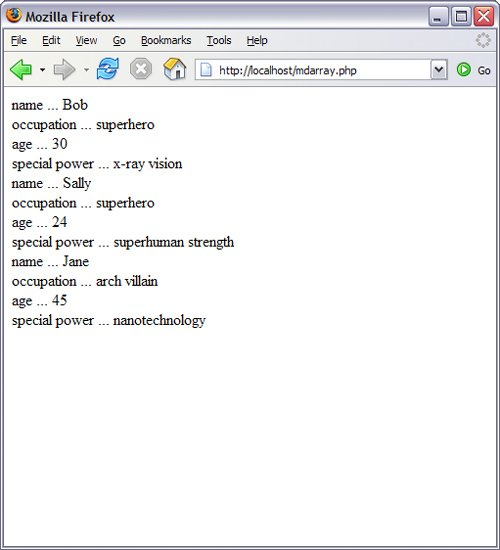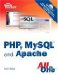# Creating Arrays

You can create an array using either the array() function or the array operator [].The array() function is usually used when you want to create a new array and populate it with more than one element, all in one fell swoop. The array operator is often used when you want to create a new array with just one element at the outset, or when you want to add to an existing array element.

The following code snippet shows how to create an array called \$rainbow using the array() function, containing all its various colors:

`\$rainbow = array("red", "orange", "yellow", "green", "blue", "indigo", "violet");`

The following snippet shows the same array being created incrementally using the array operator:

`\$rainbow[] = "red"; \$rainbow[] = "orange"; \$rainbow[] = "yellow"; \$rainbow[] = "green"; \$rainbow[] = "blue"; \$rainbow[] = "indigo"; \$rainbow[] = "violet";`

Both snippets create a seven-element array called \$rainbow, with values starting at index position 0 and ending at index position 6. If you wanted to be literal about it, you could have specified the index positions, such as in this code:

`\$rainbow = "red"; \$rainbow = "orange"; \$rainbow = "yellow"; \$rainbow = "green"; \$rainbow = "blue"; \$rainbow = "indigo"; \$rainbow = "violet";`

However, PHP does this for you when positions are not specified, and that eliminates the possibility that you will misnumber your elements, as in this example:

`\$rainbow = "red"; \$rainbow = "orange"; \$rainbow = "yellow"; \$rainbow = "green"; \$rainbow = "blue"; \$rainbow = "indigo"; \$rainbow = "violet";`

Regardless of whether you initially create your array using the array() function or the array operator, you can still add to it using the array operator. In the first line, below, six elements are added to the array, while one more element is added to the end of the array in the second line:

`\$rainbow = array("red", "orange", "yellow", "green", "blue", "indigo"); \$rainbow[] = "violet";`

The examples used in this section were of numerically indexed arrays, arguably the most common type you'll see. In the next two sections, you learn about two other types of arrays: associative and multidimensional.

#### Creating Associative Arrays

Whereas numerically indexed arrays use an index position as the key0, 1, 2, and so forthassociative arrays utilize actual named keys. The following example demonstrates this by creating an array called \$character with four elements:

`\$character = array(             "name" => "Bob",             "occupation" => "superhero",             "age" => 30,             "special power" => "x-ray vision"             );`

The four keys in the \$character array are name, occupation, age, and special power. The associated values are Bob, superhero, 30, and x-ray vision, respectively. You can reference specific elements of an associative array using the specific key, such as in this example:

`echo \$character['occupation'];`

The output of this snippet would be:

`superhero`

As with numerically indexed arrays, you can use the array operator to add to an associative array:

`\$character['supername'] = "Mega X-Ray Guy";`

This example adds a key called supername with a value of Mega X-Ray Guy.

The only difference between an associative array and a numerically indexed array is the key name; in a numerically indexed array, the key name is a number; in an associative array, the key name is a meaningful word.

#### Creating Multidimensional Arrays

The first two types of arrays hold strings and integers, whereas this third type holds other arrays. If each set of key/value pairs constitutes a dimension, a multidimensional array holds more than one series of these key/value pairs. For example, Listing 8.1 defines a multidimensional array called \$characters, each element of which contains an associative array. This may sound confusing, but it's really only an array that contains another array.

##### Listing 8.1. Defining a Multidimensional Array

 ` 1: "Bob", 5: "occupation" => "superhero", 6: "age" => 30, 7: "special power" => "x-ray vision" 8: ), 9: array( 10: "name" => "Sally", 11: "occupation" => "superhero", 12: "age" => 24, 13: "special power" => "superhuman strength" 14: ), 15: array( 16: "name" => "Jane", 17: "occupation" => "arch villain", 18: "age" => 45, 19: "special power" => "nanotechnology" 20: ) 21: ); 22: ?>`

In line 2, the \$characters array is initialized using the array() function. Lines 38 represent the first element, lines 914 represent the second element, and lines 1520 represent the third element. These elements can be referenced as \$characters, \$characters, and \$characters.

Each element consists of an associative array, itself containing four elements: name, occupation, age, and special_power.

However, if you attempt to print the master elements like so:

`echo \$characters;`

the output will be this:

`Array`

because the master element indeed holds an array as its content. To really get to the content you want, that is, the specific information found within the inner array element, you need to access the master element index position plus the associative name of the value you want to view.

Take a look at this example:

`echo \$characters['occupation'];`

It will print this:

`superhero`

If you add the following lines to the end of the code in Listing 8.1, it prints the information stored in each element:

`foreach (\$characters as \$c) {        while (list(\$k, \$v) = each (\$c)) {                echo "\$k ... \$v <br/>";        } }`

The foreach loop is concerned with the master array element, \$characters. It loops through this array and assigns the temporary name \$c to the element contained within each position. Next, we begin a while loop. This loop uses two functions to extract the contents of the inner array. First, the list() function names placeholder variables, \$k and \$v, which will be populated with the keys and values gathered from the each() function. The each() function looks at each element of the \$c array and extracts the information accordingly.

The echo statement simply prints each key and value (\$k and \$v) extracted from the \$c array using the each() function and adds a line break for display purposes. Figure 8.1 shows the result of this file, called mdarray.php.

##### Figure 8.1. Looping through a multidimensional array.Sams Teach Yourself PHP, MySQL and Apache All in One (3rd Edition)
ISBN: 0672328739
EAN: 2147483647
Year: 2004
Pages: 327